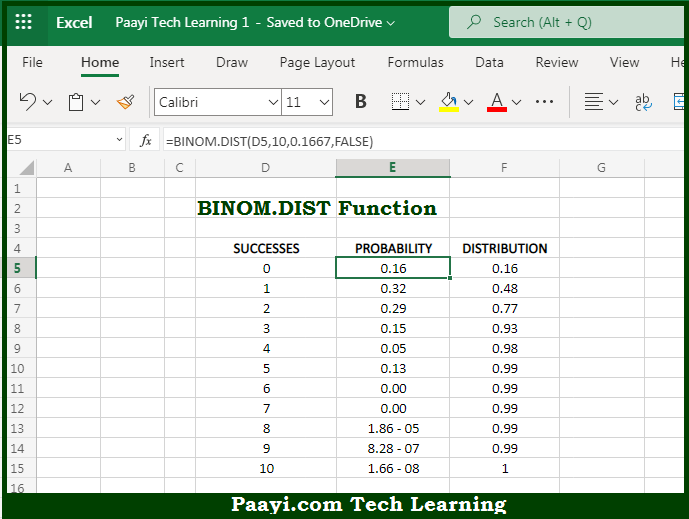# Learn How to Use Microsoft Excel BINOM.DIST Function

Written by | 0 Comments | 685 Views

In this article, you will learn how to use Microsoft Excel BINOM.DIST function and its prime function in Microsoft Excel. You will also get to know the Microsoft Excel BINOM.DIST function return value and syntax with the help of some examples.

Microsoft Excel BINOM.DIST Function

The main purpose of the Microsoft Excel BINOM.DIST function is to get the binomial distribution of the probability. That implies, with the help of BINOM.DIST function you can able to return the individual term binomial distribution probability. You can use BINOM.DIST to calculate probabilities that an event will occur a certain number of times in a given number of trials. So, with the help of BINOM.DIST function, you can able to get the binomial distribution of the probability.

Return Value of BINOM.DIST Function

The return value will be the calculated probability.

Syntax of BINOM.DIST Function

=BINOM.DIST(number-s, trials, probability-s, cumulative)

Where the arguments:

• number-s: This is the number of successes.
• trials: This is the number of independent trials.
• probability-s: This is the probability of success on each trial.
• cumulative: TRUE = cumulative distribution function, FALSE = probability mass function.

## How to Use Microsoft Excel BINOM.DIST Function?So we know that, Microsoft Excel BINOM.DIST function you can able to get the binomial distribution of the probability. That implies, with the help of BINOM.DIST function you can able to return the individual term binomial distribution probability. You can use BINOM.DIST to calculate probabilities that an event will occur a certain number of times in a given number of trials. So, with the help of BINOM.DIST function, you can able to get the binomial distribution of the probability.